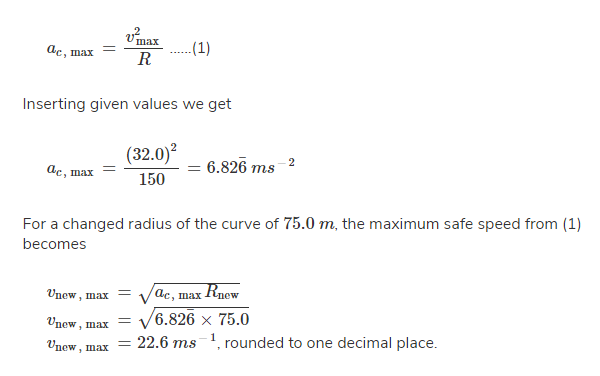Question

A certain light truck can go around a curve having a radius of $$150 m$$ with a maximum speed of $$32.0 m/s$$. To have the same acceleration, at what maximum speed can it go around a curve having a radius of $$75.0 m$$?

A
64m/sB
45m/sC
32m/sD
23m/sE
16m/sSolution

The correct option is D $$23m/s$$Friction between the tires and the flat road provides the required centripetal acceleration for the truck to negotiate the curve safely without skidding.Physics

Suggest Corrections0Similar questions
View MorePeople also searched for
View More